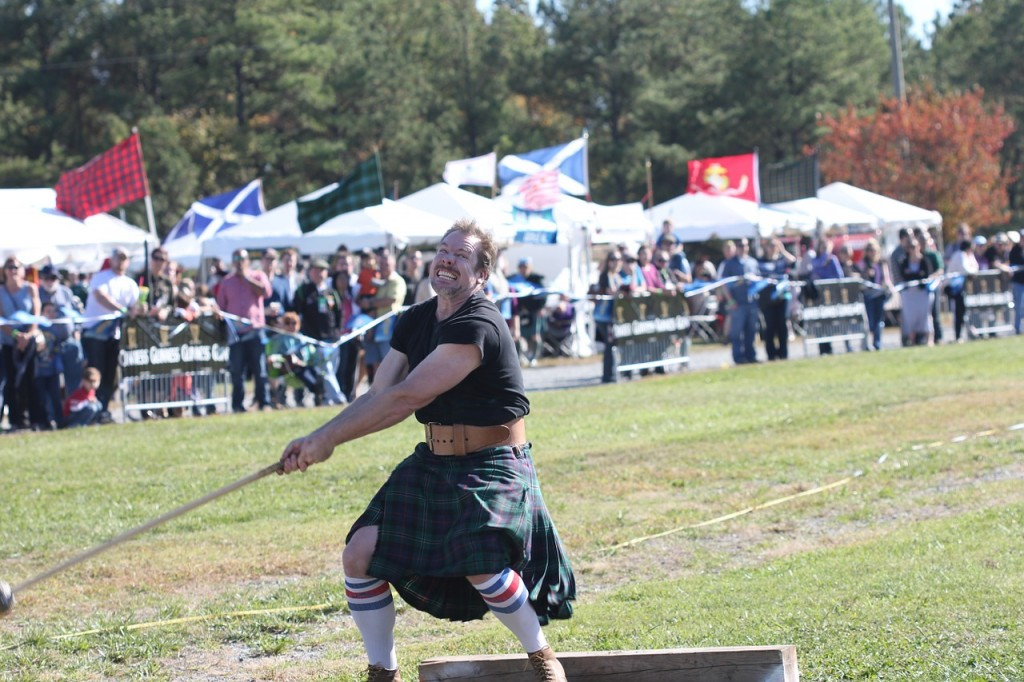# What is Circular Motion

What is Circular Motion? It is a motion of an object along the circumference of a circle. Circular motion is different from motion in a straight line, as the direction of motion is always changing. As a result, calculating quantities related to circular motion involves a different set of equations.

Circular motion is everywhere: a car traveling around a bend, a “hammer thrower” throwing their hammer and even the International Space Station orbiting Earth are all examples of circular motion.What is Circular Motion – Hammer Throw

We will look at cases where circular motion is uniform: uniform circular motion refers to circular motion, where objects have a uniform speed.

## How to Find Angular Speed

In this section, first we will learn why it is important to express angles in radians and how to convert between radians and degrees. Then, we will learn how to measure the angular speed as well as the tangential speed of an object undergoing uniform circular motion.

## How to Find Centripetal Acceleration

An object in circular motion is always accelerating. Under this section, we will look at how to define and calculate this acceleration.

## How to Calculate Centripetal Force

An object traveling in a circular path is always experiencing a force directed towards the centre of the circular path. This force is called the centripetal force. Here, we will look at how centripetal force is defined and how to calculate the centripetal force for objects in uniform circular motion in horizontal circles. We also look at centripetal forces in conical pendulums, in vehicles traveling on banked roads, and in tilted aeroplanes.

## How to Solve Vertical Circular Motion Problems

When objects travel in vertical circles, the forces acting on the particle are always changing. This section explains how to calculate centripetal forces in several such cases, including when objects travel in vertical circles at constant speeds and when objects travel at varying speeds. We will also look at how a bucket of water may be swung overhead without the water spilling out. We will also learn how the principles of the circular motion is used in “loop the loop” roller coaster rides.